# Shapes of Orbitals and Electronic ConfigurationShapes of Orbitals and Electronic Configuration

Shapes of Orbitals:

(i) s-orbitals: For s-orbitals, Ɩ = 0 and hence m = 0. So, there is only one possible orientation for s-orbitals. They are spherically symmetrical. The plots of probability density (ψ2) against distance from the nucleus (r) for 1s and 2s atomic orbitals are as follows: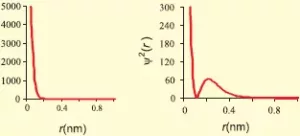For 1s orbital, the probability density is maximum at the nucleus and it decreases with an increase in r. But for 2s orbital, the probability density first decreases sharply to zero and again starts increasing. After reaching a small maximum it decreases again and approaches zero as the value of r increases. The region where the probability density (ψ2) reduces to zero is called nodal surface or node.
In general, for an ns-orbital, there are (n – 1) nodes.All the s-orbitals are spherically symmetrical and their size increases with an increase in n.
s-orbital has a spherical shapeShapes of Orbitals and Electronic Configuration

(ii) p-orbitals: For p-orbitals, Ɩ = 1 and mƖ = -1, 0, +1. i.e., there are three possible orientations for p orbitals. So, there are 3 types of p-orbitals – px, py, and pz. Each p orbital consists of two lobes. The probability density function is zero on the plane where the two lobes touch each other.
The size, shape, and energy of the three orbitals are identical. They differ only in the orientation of the lobes. For px orbital, the lobes are along the x-axis, for py, they are along the y-axis, and for pz, they are along the z-axis. All the p-orbitals have a dumb-bell shape.
Number of radial nodes = n – Ɩ – 1
Number of angular nodes = Ɩ
Total number of nodes = n-1
The diagrams for three 2p orbitals are as follows: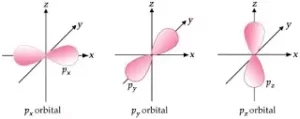(iii) d-orbitals:
For d-orbitals, Ɩ = 2 and mƖ = -2, -1, 0, +1 and +2. i.e., there are five possible orientations for d orbitals. So there are 5 types of d-orbitals. They are dxy, dyz, dzx, dx2-y2, and dz2. The shapes of the first four d-orbitals are double dumb-bell and that of the fifth one, dz2, is dumb-bell having a circular collar in the xy-plane. The five d- orbitals have equivalent energies. For d-orbitals, the number of radial nodes is 2 and the total number of nodes is n-2. Boundary surface diagrams for d-orbitals are as follows: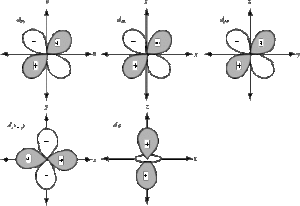(iv) f-orbitals:
For f-orbitals, Ɩ = 3 and mƖ = -3, -2, -1, 0, +1, +2 and +3. i.e., there are seven possible orientations for f orbitals. So there are 7 types of f-orbitals. They are fx3, fy3, fz3, fx(y2-z2), fy(z2-x2), fz(x2-y2) and fxyz. They have diffused shapes.

Shapes of Orbitals and Electronic Configuration

Electronic Configuration of Atoms: The arrangement of electrons in various orbitals is called the electronic configuration. This arrangement is obtained on the basis of following rules,

(i) Aufbau principle:  Electrons are filled in the various orbitals in the increasing order of their energies, i.e., orbital having lowest energy will be filled first and the orbital having highest energy will be filled last.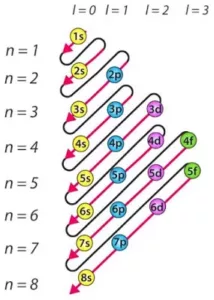This rule has two sub rules:
a) The various orbitals are filled in the increasing order of their (n+Ɩ)
b) If two orbitals have the same (n+Ɩ) values, the orbital with the lower n value is filled first this rule is called Bohr-Bury rule. The increasing order of orbitals is asfollows:
1s < 2s < 2p < 3s <3p < 4s <3d < 4p <5s < 4d <5p < 6s < 4f < 5d < 6p < 7s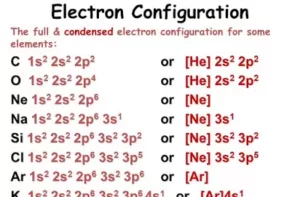Shapes of Orbitals and Electronic Configuration

(ii)  Pauli’s Exclusion Principle: No two electrons in an atom can have all the four quantum numbers the same.  It can also be stated as – An orbital can have a maximum of two electrons and they must be of opposite spin.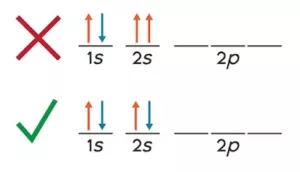(iii) Hund’s Rule of Maximum Multiplicity: No electron pairing takes place until each orbital is first singlifilled, e.g., N (7) has electronic configuration 1s2 2s2, 2px1 2py1 2pz1 according to Hund’s rule.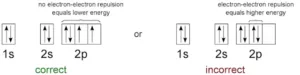Degenerate orbitals: Orbitals having the same energy are called degenerate orbitals.

Shielding Effect or Screening Effect:  Due to the presence of electrons in the inner shells, the electron in the outer shell will not experience the full positive charge on the nucleus. So due to the screening effect, the net positive charge experienced by the electron from the nucleus is lowered and is known as an effective nuclear charge.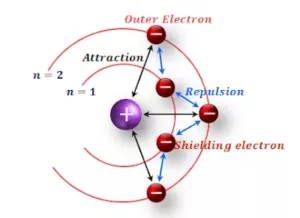Shapes of Orbitals and Electronic Configuration

Stability of Completely Filled and Half Filled Subshells:
(i) For atoms having half-filled or completely filled electronic configurations have extra stability compared to other atoms. This is due to their symmetrical distribution of electrons and greater exchange energy. For example, the electronic configuration of Cr is [Ar] 3d54s1 and not 3d44s2. This is because d5 represents a half-filled configuration and has extra stability. Similarly for Cu the electronic configuration is [Ar] 3d104s1 and not 3d94s2.Another factor that explains the stability of half-filled orbitals is exchange energy. More the number of exchanges more is the stability.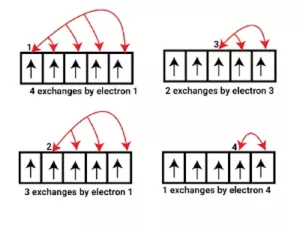Electronic Configuration of Elements From Hydrogen to Zinc: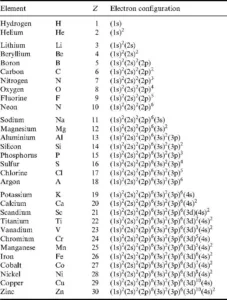Electronic Configuration Along with Orbital Diagram: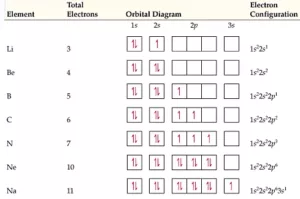Shapes of Orbitals and Electronic Configuration